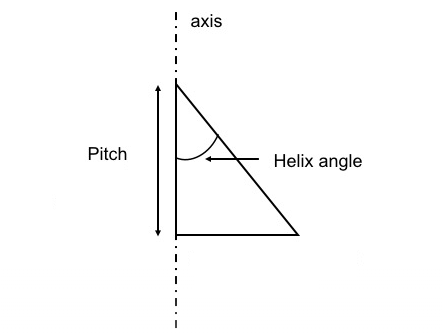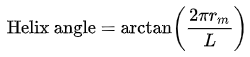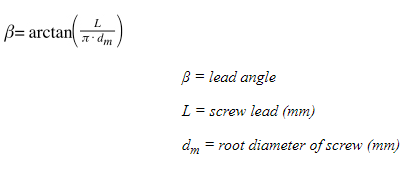> > What is Helix Angle & How to Calculate It – Helix Angle vs Lead Angle, What’s the Difference | CNCLATHING
> > What is Helix Angle & How to Calculate It – Helix Angle vs Lead Angle, What’s the Difference | CNCLATHING

# What is Helix Angle & How to Calculate It – Helix Angle vs Lead Angle, What’s the Difference | CNCLATHING

2021.4.19

A helix angle or spiral angle is a critical feature of a twist drill, which usually used to cut materials in CNC services. The selection of helix angles matters a lot when working with different materials. Follow us to understand what is helix angle, drill helix angle selection, helix angle calculation and the difference between lead angle and helix angle.

## What is Helix Angle?

The shape formed by the threads of a screw is called a helix. Screws, helical gears and worm gears are common mechanical components where you can find helical threads. If you take a single turn of the helix and unwind it into a straight line, the length of the straight line can be calculated as the circumference of a circle, while a helix angle is defined as the angle between this unwound circumferential line and the pitch of the helix. Generally, the helix angle is the geometric complement of the lead angle.What is helix angle in drill? The helix angle of a drill bit is defined as the angle between the screw flight of a helix and any line perpendicular to the screw axis or pitch. Different helix angles on drill bits are suitable for different applications, for example, the drill bit with a high helix angle is more effective for cutting soft and long-chipping materials, as a high helix angle improves penetration efficiency and increases the force pushing the material out of the hole being bored, also decrease the helical cross-sectional area and weaken the body of the drill. On the contrary, the drill bit with a lower helix angle is suitable for drilling hard and short-chipping materials.

## How to Calculate Helix Angle? - Helix Angle Formula

The formula Helix angle = Atan (Lead of Screw/Circumference of Screw) or α= atan(L/C) is used to calculate the Helix Angle, which is represented by α symbol.

Note: The Atan or arc tangent function is to calculate the value (in radians) of the angle whose tangent equals a specified number (the inverse of the Tan function).

Lead of Screw: the linear travel the nut makes per one screw revolution.

Circumference of Screw: calculated by measuring the diameter of the screw and multiplying by pi.

## Helix Angle vs Lead Angle - Difference Between Lead Angle and Helix Angle

The helix angle is basically a concept applied to helical gears and bevel gears. The acute angle between the tangent line of the cylindrical helix and the straight generatrix of cylindrical surface passing through the tangent point, and the acute angle between the tangent line of the conical helix and the straight generatrix of conical surface passing through the tangent point are all called helix angle. Generally speaking, the helix angle is actually the helix angle on the indexing circle. Lead angle is generally described in thread and worm. It is also called “thread rising angle” for the thread. It is the angle between tangent line of the helix on a pitch diameter cylinder or pitch diameter cone and a plane perpendicular to the thread axis. The terms “lead angle” and “helix angle” are often used interchangeably, but the helix angle is the angle between the helix of the thread and a line parallel to the axis of rotation. Helix and lead angle are complementary; their sum must equal 90 degrees.

1. Feature/Definition:

The helix angle references the axis of the cylinder, distinguishing it from the lead angle, which references a line perpendicular to the axis. The helix angle is the angle opposite the lead angle.

2. Uses

Helix angle: helical gear and worm gear, etc. Generally, the helix angle is used to indicate the inclination degree of gear teeth.

Lead angle: used for referring to screw geometry.

3. Calculation

Helix angle formula: